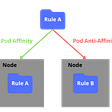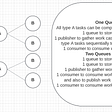# Python Prerequisites for Data Science Part I : Python Data Structures

• Integer : Represented by the keyword ‘int’
• Float : Represented by the keyword ‘float’
• Strings : Represented by the keyword ‘str’
• Boolean : Represented by the keyword ‘bool’
• Null : Represented by the keyword ‘NoneType’

# Lists:

## Initialization and Definition:

`# First Method to Initialize Listl = []# Second Method to Initialize Listl = list()# Creating a list of heterogenous datatypesl = [3, 4.5, 5.67, "Hello", None, True, 0.45, 'False']`

## Indexing and Slicing:

• l[i] : Gives the ith element
• l[-i] : Gives the ith element starting from the end of the list
• l[a:b] : Gives a sublist with elements from ath element to (b-1)th element
• l[a:] : Gives a sublist with elements after ath element (inclusive)
• l[:b] : Gives a sublist with elements before bth element (exclusive)
`print(l) #Gives output 3print(l[-1]) #Gives output 'False'print(l[2:7]) #Gives output [5.67, "Hello", None, True, 0.45]print(l[4:]) #Gives output ["Hello", None, True, 0.45, 'False']print(l[:5]) #Gives output [3, 4.5, 5.67, "Hello", None]`

## Object Assignment in Python:

`#Assigning two different variables with same listx = y = [2, 4, 5, 7]#Changing an element from the list variable xx = 8 #Changes the 3rd element of the list to 8#Viewing the contents of list variable yprint(y) #Gives output: [2, 4, 8, 7]`
`#First Method: (Using slicing to create a new list)y = x[:] #Copies all content of x and creates a new list#Second Method: (Using built-in copy() method for objects)y = x.copy() #Creates a list by copying the contents of x`

## Built-In Methods:

`#Creating a list of values to apply operationsl = [2.5, 7.8, 12.4, 0.45, 12.0, 5.67, 0.45]print(min(l)) #Gives output 0.45print(max(l)) #Gives output 12.4print(del(l)) #Gives output [2.5, 7.8, 0.45, 12.0, 5.67, 0.45]print(l.index(7.8)) #Gives output 1print(l.count(0.45)) #Gives output 2`

# Strings:

## Initialization and Definition:

`#Creating a string (First Method)s = "Hello"#Creating a string (Second Method)s = 'Hello'`

## Trying out String Manipulation:

`#Changing the 2nd character of the string to 'a's = 'a'#Outputs TypeError: 'str' object does not support item assignment`

## Built-In Methods:

`#Creating a string to check different built-in methodss = "Hello World*"print(s.lower()) #Outputs "hello world*"print(s.upper()) #Outputs "HELLO WORLD*"print(s.capitalize()) #Outputs "Hello world*"print(s.split()) #Outputs ["Hello", "World*"]print(s.strip("*")) #Outputs "Hello World"print(s.replace("*","")) #Outputs "Hello World" `

# Tuples:

## Initialization and Definition:

`#First Method to Initializet = ()#Second Method to Initializet = tuple()#Creating a tuplet = (3, 4.5, "Hello")`

## Unpacking Values:

`#Assigning values of tuples to three different variablesx, y, z = tprint(x) #Gives output 3print(y) #Gives output 4.5print(z) #Gives output "Hello"`

## Indexing and Slicing:

`print(t) #Gives output 3print(t[:2]) #Gives output (3, 4.5)`

# Sets:

## Initialization and Definition:

`#Initializing a sets = set()#Creating a set of valuess = {1, 2, 3, 3, 5, 6, 7, 8, 8, 8, 9}print(s) #Gives output {1,2,3,5,6,7,8,9}`

## Set Operations:

`#Creating two sets to perform set operationsA = {1,2,3,4,5}B = {3,4,5,6,7}print(A.union(B)) #Gives output {1,2,3,4,5,6,7}print(A.intersection(B)) #Gives output {3,4,5}print(A.difference(B)) #Gives output {1,2}print(A.symmetric_difference(B)) #Gives output {1,2,6,7}`

# Dictionaries:

`#Initializing a dictionary (First Method)d = {}#Initializing a dictionary (Second Method)d = dict()#Creating a dictionaryd = {'a':2, 'b':3, 'c':4}`

## Key Immutability:

`#Creating a dictionary with string and tuple as keysd = {"gridsize":(3,3), (2,3):7} #Works perfectly fine#Creating a dictionary with list as keyd = {"gridsize":(3,3), [2,3]:7} #Gives TypeError: unhashable type: 'list'`

## Indexing and Mutability:

`#Creating a dictionaryd = {'a':3, 'b':4, 'c':5} #Indexing value of 'b' in dictionaryprint(d['b']) #Gives output 4#Performing assignment via indexingd['b'] = 6print(d) #Gives output {'a':3, 'b':6, 'c':5}`

## Built-In Methods:

`d.update({'d':7, 'e':8})print(d) #Gives output {'a':3, 'b':6, 'c':5, 'd':7, 'e':8}print(d.keys()) #Gives output dict_keys(['a','b','c','d','e','f'])print(d.items()) #Gives output dict_items([('a', 3), ('b', 6), ('c', 7), ('d', 9), ('e', 6), ('f', 10)])`

# That’s it for this Part

--

--

--

## More from Hassan Farid

Python Developer | Data Science

Love podcasts or audiobooks? Learn on the go with our new app.

## Kubernetes Scheduling Abridged## Groupcache instrumented by OpenCensus## Application Reliability with APM## Project progress 03.06.2022## Cyber Research #24## On queue and queue designs (in RPA)## Kubernetes vs Docker: Which is better## Defect Management and Life Cycle## Hassan Farid

Python Developer | Data Science

## Learn how to handle timestamps and timezones in Python in 2 minutes## LightGBM Starter Code## Machine Learning in Python — Amazon Reviews## Python for Data Science — Session Codest